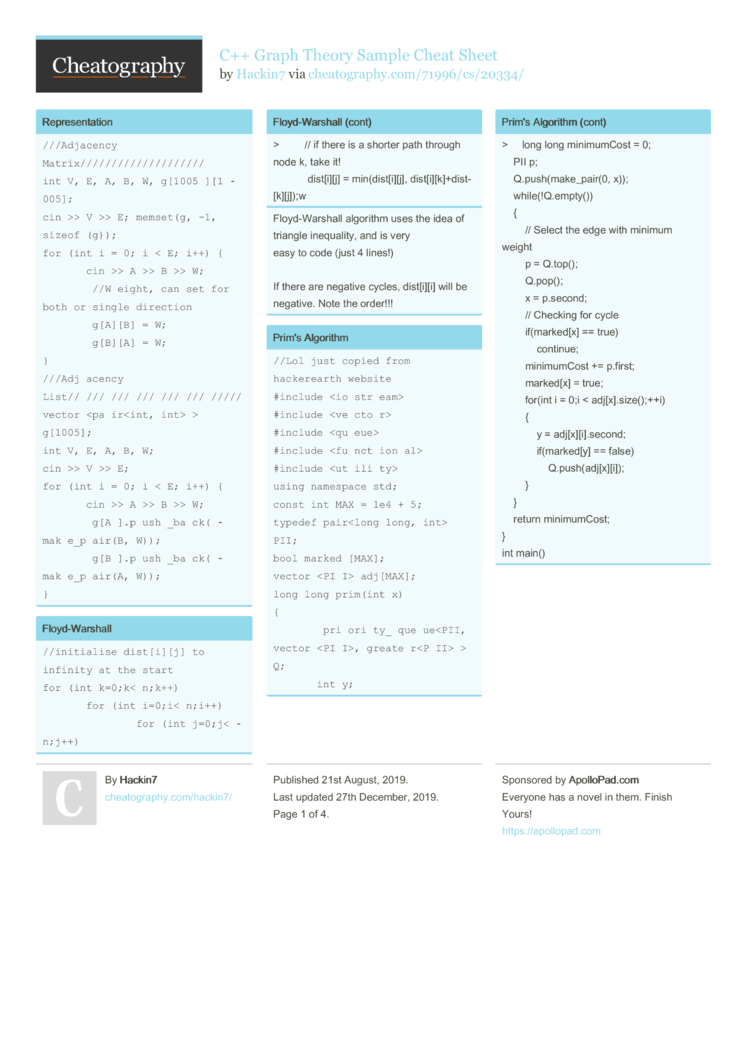# C++ Graph Theory Sample Cheat Sheet by Hackin7

Some sample graph theory code that can be used

### Repres­ent­ation

 ```///Adjacency Matrix//////////////////// int V, E, A, B, W, g; cin >> V >> E; memset(g, -1, sizeof(g)); for (int i = 0; i < E; i++) {     cin >> A >> B >> W;     //Weight, can set for both or single direction     g[A][B] = W;     g[B][A] = W; } ///Adjacency List////////////////////// vector > g; int V, E, A, B, W; cin >> V >> E; for (int i = 0; i < E; i++) {     cin >> A >> B >> W;     g[A].push_back(make_pair(B, W));     g[B].push_back(make_pair(A, W)); }```

### Floyd-­War­shall

 ```//initialise dist[i][j] to infinity at the start for (int k=0;k
Floyd-­War­shall algorithm uses the idea of triangle inequa­lity, and is very
easy to code (just 4 lines!)

If there are negative cycles, dist[i][i] will be negative. Note the order!!!

 ```vector g; queue > q; int dist; fill(dist, dist+1000005, -1); while (!q.empty()) {     int v = q.front().first;     int d = q.front().second;     q.pop();     if (dist[v] != -1) continue; //Visited     dist[v] = d;     for (int i = 0; i < g[v].size(); i++) {         q.push(make_pair(g[v][i], d+1));     } }```
Time Comple­xity: O(|V| + |E|)
Space Comple­xity: O(b^d)
where d is the depth of the graph and b is the branching factor.

BFS is more suitable when the goal is close to the source, BFS is still faster in such cases.

We can use this algorithm to find the shortest path in a grid/u­nwe­ighted
graph

### Bellma­n-Ford

 ```dist[s]=0; //dist all others = INF for (int i=0; i
Solves the Single Source Shortest Path (SSSP) problem. (shortest path from one node (source) to all other nodes)
Can be used with negative edges, Run the algorithm twice to detect for negative cycles

Time Comple­xity: O(VE)
Space Comple­xity: O(V)

### Depth First Search

 ```bool vis[N]; vector adjList[N]; void dfs(int node) {     if (vis[node]) return;     vis[node] = true;     for (int a = 0; a < (int)adjList[node].size(); ++a)         dfs(adjList[node][a]); } ///Iterative//////////////////////////////// bool vis[N]; vector adjList[N]; stack S; while (!S.empty()) {     int node = S.top();     S.pop();     if (vis[node]) continue;     vis[node] = true;     for (int a = 0; a < (int)adjList[node].size(); ++a)         S.push(adjList[node][a]); }```
DFS uses O(d) space, where d is the depth of the graph

DFS is not suited for infinite graphs.

Some applic­ations of DFS include:
1. Topolo­gical Ordering (covered later)
2. Pre-/I­n-/­Pos­t-order numbering of a tree
3. Graph connec­tivity
4. Finding articu­lation points
5. Finding bridges

### Dijkstra's Algorithm

 ```vector > adjList; // node, weight priority_queue, vector >, greater > > pq; // distance, node int dist; memset(dist, -1, sizeof(dist)); pq.push(make_pair(0, source)); dist = 0; while(!pq.empty()){     pair c = pq.top();     pq.pop();     if(c.first != dist[c.second]) continue;     for(auto i : adjList[c.second]){         if(dist[i.first] == -1 || dist[i.first] > c.first + i.second){             dist[i.first] = c.first + i.second;             pq.push(make_pair(dist[i.first], i.first));         }     } }```
Time Complexity of our implem­ent­ation: O(E log E)
Space Comple­xity: O(V+E)

Solves the Single Source Shortest Path (SSSP) problem. Means shortest path from one node to all other nodes. Cannot be used with negative edges as it runs too slow
Especially cannot be used with negative cycles2 Pages
//media.cheatography.com/storage/thumb/hackin7_c-graph-theory-sample.750.jpg

PDF (recommended)